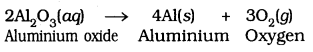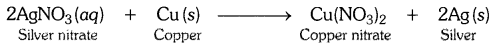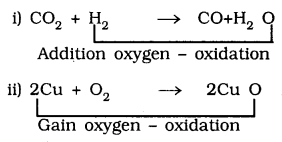# KSEEB SSLC Class 10 Science Solutions Chapter 1 Chemical Reactions and Equations

KSEEB SSLC Class 10 Science Solutions Chapter 1 Chemical Reactions and Equations are part of KSEEB SSLC Class 10 Science Solutions. Here we have given Karnataka SSLC Class 10 Science Solutions Chapter 1 Chemical Reactions and Equations.

## Karnataka SSLC Class 10 Science Solutions Chapter 1 Chemical Reactions and Equations

### KSEEB SSLC Class 10 Science Chapter 1 Intext Questions

Text Book Part I Page No. 6

Question 1.
Why should a magnesium ribbon be cleaned before burning in air?
Magnesium metal is highly reactive. In stored conditions, it reacts with oxygen to form magnesium oxide over its outer layer. To remove this layer and to expose the underlying metal into air, the magnesium ribbon is cleaned by sandpaper.

Question 2.
Write the balanced equation for the following chemical reactions.
i) Hydrogen + Chlorine ➝ Hydrogen Chloride
H2(g) + Cl2(g) ➝ 2HCl(g)

ii) Barium chloride + Aluminium sulphate ➝ Barium sulphate + Aluminium chloride
3BaCl2(s) + Al2(SO4)3(s) ➝ 3BaSO4 + 2Al2Cl3(s)

iii) Sodium + water ➝ Sodium hydroxide + Hydrogen
2Na(s) + 2H2O(l) ➝ 2NaOH(aq) + H2(g)

Question 3.
Write a balanced chemical equation with state symbols for the following reactions.
i) Solutions of barium chloride and sodium sulphate in water react to give insoluble barium sulphate and the solution of sodium chloride.
Balanced chemical equations with state symbols for the required reactions are as follows:

1. BaCl2(aq) + Na2SO4(aq) → BaSO4(s) + 2NaCl(aq)
2. NaOH(aq) + HCl(aq) + NaCl(aq) + H2O(l)

Text Book Part I Page No. 10

Question 1.
A solution of a substance ‘X’ is used for whitewashing.
i) Name the substance ‘X’ and write its formula.
The substance X is calcium Hydroxide. Its formula is Ca(OH)2

ii) Write the reaction of the substance ‘X’ named in (i) above with water.
Cao + H2O ➝ Ca(OH)2

Question 2.
Why is the amount of gas collected in one of the test tubes in ActivityWater (H2O) contains two parts of hydrogen and one part of oxygen. The ratio of water components i.e., hydrogen and oxygen is 2:1. Therefore, the amount of hydrogen and oxygen produced after water electrolysis is in a ratio of 2:1. This is why during electrolysis the amount of gas collected in hydrogen’s test tubes is double the amount collected in the oxygen’s test tube.

1. Take a plastic mug. Drill two holes at its base and fit rubber stoppers in these holes. Insert carbon electrodes in these rubber stoppers as shown in Figure.
2. Connect these electrodes to a 6-volt battery.
3. Fill the mug with water such that the electrodes are immersed. Add a few drops of dilute sulphuric acid to the water.
4. Take two test tubes filled with water and invert them over the two carbon electrodes.
5. Switch on the current and leave the apparatus undisturbed for some time.
6. You will observe the formation of bubbles at both electrodes. These bubbles displace water in the test tubes.
7. Is the volume of the gas collected the same in both the test tubes?
8. Once the test tubes are filled with the respective gases. Remove them carefully.
9. Test these gases one by one by bringing a burning candle close to the mouth of the test tubes.

Caution:

1. This step must be performed carefully by the teacher.
2. What happens in each case?
3. Which gas is present in each test tube?

Text Book Part I Page No. 13

Question 1.
Why does the colour of copper sulphate solution change when an iron nail is dipped in it?
Iron is more reactive than copper. When an iron nail is dipped in copper sulphate solution, iron forms its sulphate (iron sulphate) solution by displacing copper of copper sulphate. The colour of iron sulphate is green. So, colour change in solution appears.

Question 2.
Give an example of a double displacement reaction other than the one given in Activity 1.10.
2KBr(aq)+BaI2(aq) ➝ 2KI(aq) + BaBr2 (aq)

Question 3.
Identify the substances that are oxidised and the substances that are reduced in the following reactions.
i) 4Na(s) + O2(g) ➝ 2Na2O(s) 4Na(s)
Sodium is oxidized because it gets oxygen and forms sodium oxide.

ii) CuO(s) + H2(g) ➝ Cu(s) + H2O(l)
Copper oxide reduced to copper H2 changes to water.

### KSEEB SSLC Class 10 Science Chapter 1 Textbook Exercises

Question 1.
Which of the statements about the reaction below are incorrect?
2PbO(s) + C(s) ➝ 2Pb(s) + CO2(g)
b) Carbon dioxide is getting oxidised.
c) Carbon is getting oxidised.
d) Lead oxide is getting reduced.
i) (a) and (b)
ii) (a) and (c)
iii) (a), (b) and (c)
iv) all
(i) (a) and (b).
Lead is getting reduced. & Carbon dioxide is getting oxidised.

Question 2.
Fe2O3 + 2Al ➝ Al2O3 + 2Fe
The above reaction is an example of a
a) combination reaction
b) double displacement reaction.
c) decompoistion reaction.
d) displacement reaction.
(d) The reaction is an example of a displacement reaction.

Question 3.
What happens when dilute hydrochloric acid is added to iron fillings? Tick the correct answer.
a) Hydrogen gas and iron chloride are produced.
b) Chlorine gas and iron hydroxide are produced.
c) No reaction takes place.
d) Iron salt and water are produced.
(a) Hydrogen gas and iron chloride are produced.
Fe(s) + 2HCl(aq) ➝ FeCl2(aq) + H2l

Question 4.
What is a balanced chemical equation? Why should chemical eqations be balanced?
The total mass of the elements present in the products of a chemical reaction has to be equal to the total mass of the elements present in the reactants. In other words, the number of atoms of each element remains the same, before and after a chemical reaction. This is called Balancing equation. We must balance the chemical equation, otherwise it becomes skeletal chemical equation.

Question 5.
Translate the following statements into chemical equations and then balance them.
(a) Hydrogen gas combines with nitrogen to form ammonia.
(b) Hydrogen sulphide gas burns in air to give water and sulphur dioxide.
(c) Barium chloride reacts with aluminium sulphate to give aluminium chloride and a precipitate of barium sulphate.
(d) Potassium metal reacts with water to give potassium hydroxide and hydrogen gas.
(a) 3H2(g) + N2(g) → 2NH3(g).
(b) 2H2S(g) + 3O2(g) → 2H2O(l) + 2SO2(g).
(c) 3BaCl2(aq) + Al2(SO4)3(aq) → 2AlCl3(aq) + 3BaSO4(s).
(d) 2K(s) + 2H2O(l) → 2KOH(aq) + H2(g).

Question 6.
Balance the following chemical equations.
a) HNO3 + Ca(OH)2 ➝ Ca(NO3)2 + H2O
b) NaOH + H2SO4 ➝ Na2SO4 + H2O
c) NaCl + AgNO3 ➝ AgCl + NaNO3
d) BaCl2 + H2SO4 ➝ BaSO4 + HCl
a) 2HNO3 + Ca(OH)2 ➝ Ca(NO3)2 + 2H2O
b) 2NaOH + H2SO4 ➝ Na2SO4 + 2H2O
c) NaCl + AgNO3 ➝ AgCl + NaNO3
d) BaCl2 + H2SO4 ➝ BaSO4 + 2HCl

Question 7.
Write the balanced chemical equations for the following reactions.
a) Calcium hydroxide + Carbon dioxide ➝ Calcium carbonate + Water
b) Zinc + Silver nitrate ➝ Zinc nitrate + Silver
c) Aluminium + Copper chloride ➝ Aluminium chloride + Copper
d) Barium chloride + Potassium sulphate ➝ Barium sulphate + Potassium chloride
a) Ca(OH)2 + CO2 ➝ CaCO3 + H2O
b) Zn + 2AgNO3 ➝ Zn(NO3)2 + 2Ag
c) 2Al + 3CuCl2 ➝ AlCl3 + 3Cu
d) BaCl2 + K2SO4 ➝ BaSO4 + KCl

Question 8.
Write the balanced chemical equation for the following and identify the type of reaction in each case.
a) Potassium bromide(aq) + Barium iodide(aq) ➝ Potassium iodide (aq) + Barium bromide(s)
b) Zinc carbonate(s) ➝ Zinc oxide(s) + Carbon dioxide(g)
c) Hydrogen(g) + Chlorine(g) ➝ Hydrogen chloride(g)
d) Magnesium(s) + Hydrocholoric acid(aq) ➝ Magnesium chloride(aq) + Hydrogen(g)
a) 2KBr(aq) + Bal2(aq) ➝ 2KI(aq) + BaBr2(s) ➝ double displacement reaction.
b) ZnCO3(s) ➝ ZnO(s) + CO2(g) ➝ decompoistion reaction.
c) H2(g) + Cl2(q) ➝ 2HCl(g) ➝ combination reaction
d) Mg(s) + 2HCl(aq) —> MgCl2(aq) + H2(g) ➝ displacement reaction.

Question 9.
What does one mean by exothermic and endothermic reactions? Give examples.

1.  Reactions in which heat is released along with the formation of products are called exothermic chemical reactions.
Eg: CH4(g)+2O2(g) ➝ CO2(g) + 2H2O(g)
2.  Reactions in which energy is absorbed are known as endothermic reactions.
6CO2 + 6H2O(l) ➝ C6H12O6(aq) + 6O2(g)

Question 10.
Why is respiration considered an exothermic reaction? Explain.
We get from the food we eat. During digestion complex molecules of food are broken into simpler molecule such as glucose. This glucose combines with oxygen in the cells of our body and provides energy. Therefore respiration is considered an exothermic reaction.

Question 11.
Why are decomposition reactions called the opposite of combination reactions? Write equations for these reactions.
Decomposition reactions involve breaking down of compounds to form two or more substances. These reactions require energy to proceed. Thus, they are the exact opposite of combination reactions in which two or more substances combine to give a new substance.

Examples:

1. ZnCO3(s) → ZnO(s) + CO2(g); Decomposition reaction.
2. H2(g) + Cl2(g) → 2HCl(g); Combination reaction.

In the first equation, since ZnCO3 is broken down into ZnO and CO2 it is a decomposition reaction. In the second equation, H2 and Cl2 combine to give a new substance HCl. Therefore, it is a combination reaction.

Question 12.
Write one equation each for decomposition reactions where energy is supplied in the form of heat, light or electricity.
Ans:
a) Thermal decompositionb) Decomposition by lightc) Decomposition by electricityQuestion 13.
What is the difference between displacement and double displacement reactions Write equations for these reactions.
In a displacement reaction, a more reactive element displaces a less reactive element from a compound.

A + BX → AX + B; where A is more reactive than B.
In a double displacement reaction, two atoms or a group of atoms switch places to form new compounds.
AB + CD → AD + CB
For example:
Displacement reaction:
CuSO4(aq) + Zn(s) → ZnSO4(aq) + Cu(s)

Double displacement reaction:
Na2SO4(aq) + BaCl2 (aq) → BaSO4(s) + 2NaCl(aq)

Question 14.
In the refining of silver, the recovery of silver from silver nitrate solution involved displacement by copper metal. Write down the reactions involved.Question 15.
What do you mean by a precipitation reaction? Explain by giving examples.
Any reaction that produces a precipitate can be called a precipitation reaction.
Eg:In this reaction Barium chloride obtained as precipitate.
The white precipatate of BaSO4 is formed by the reaction of SO42- and Ba2+. The other product is formed is Sodium Chloride which remains in the solution.

Question 16.
Explain the following in terms of gain or loss of oxygen with two examples each.
a) Oxidation (Gain of Oxygen)In equation (i) From H2, H2O is oxidised. In eqn (ii) From Cu, CuO is oxidised
b) Reduction is loss of Oxygen:In eqn (i) CO2 is reduced to CO. in eqn (ii) CuO is reduced to Cu.

Question 17.
A shiny brown coloured element ‘X’ on heating in air becomes black in colour. Name the element ‘X’ and the black coloured compound formed.
‘X’ means copper (Cu) and black coloured compound copper oxideQuestion 18.
Why do we apply paint on iron articles?
Iron articles are painted to prevent them from rusting. When painted, the contact of iron articles with atmospheric moisture and the air is cut off. Hence, rusting is prevented.

Question 19.
Oil and fat containing food items are flushed with nitrogen. Why?

Oil and fat containing food items are flushed with nitrogen to prevent the items from getting oxidised which may result in rancidity of such products. When fats and oils are oxidised, they become rancid and their smell and taste change. Nitrogen provides an inert atmosphere for them.

Question 20.
Explain the following terms with one example each.
a) Corrosion
b) Rancidity

(a) Corrosion:
When a metal is attacked by substances around it such as moisture, acids etc. it gets corroded and the process is called corrosion. For example, rusting of iron products.

(b) Rancidity:
The Process in which fats and oils or food products made from fats or oils get oxidised resulting in a change of smell and the taste is called rancidity. For example, food items made from oil like chips becomes rancid if kept open for some time.

To prevent rancidity antioxidants (which prevent oxidation) are added to food containing fats and oils. Rancidity can also be prevented by flushing out oxygen with a gas like nitrogen. For example packets of food items like chips are flushed with nitrogen so that those can be used even after long duration.

### KSEEB SSLC Class 10 Science Chapter 1 Additional Questions and Answers

Question 1.
How do you determine whether a chemical reaction has taken place?
Following observations helps us to determine whether a chemical reaction has taken place

1.  Change in state
2.  Change in colour
3.  evolution of a gas
4.  Change in temperature.

Question 2.
Write the Balanced equation for the reaction of iron with steam.
3Fe(s) + 4H2O(g) ➝ Fe3O4(s) + 4H2(g)

Question 3.
What is quicklime? Write one use of quicklime.